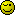# PHP Gotcha #3: PHP's variable copy and cloning behavior

Consider the following code.

``````
\$a = new stdClass();
\$a->foo = 'foo';
\$b = \$a;
echo \$b->foo; // 'foo' of course.
\$a->foo = 'bar';
echo \$b->foo; // 'bar'

``````

In PHP, objects are always passed by reference. If you don’t want this to happen, you must clone them. That said, this isn’t that different from other languages like JavaScript. The gotcha is that PHP only behaves this way with objects - other scalars don’t maintain equivalency after assignment. Try the following example:

``````
\$a = 'foo';
\$b = \$a;
\$a = 'bar';
echo \$b; // foo;

``````

You can pass by reference with the & operator though.

``````
\$a = 'foo';
\$b =& \$a;
\$a = 'bar';
echo \$b; // bar;

``````

Arguments to functions can be passed by reference or have their returns [url=http://php.net/manual/en/language.references.pass.php]received by reference. There are valid reasons for doing either of these but use this functionality with caution - misused it can lead to hard to debug code. Of particular concern is the practice of receiving a variable by reference, then changing it’s value. As a general rule a function shouldn’t change the program state in any way other than to issue its return, nor should it have any internal state. However, the technique of receiving by reference and then operating on the passed variables is used in the language itself with several of the array sorting methods ([fphp]sort[/fphp], [fphp]arsort[/fphp], etc). This is done to conserve memory.

Indeed this is a pretty good one to get caught out on if you’re new to PHP, a while back I was helping someone with pass by reference and asked them to work out what I did with the following, safe to say they couldn’t work it out.

``````\$a      = 'hello';
\$\$a     = 'world';

function change(&\$input) {
\$input = 'world';
}

echo '\$a  = ' . \$a . '<br>';
echo '\$\$a = ' . \$\$a . '<br><br>';

change(\$a);

echo \$a . ' ' . \$hello;
``````

\$\$ is something you can do in PHP, but for the life of me I can’t figure out why you would ever want to. I mean in the global namespace \$\$foo is the same as \$GLOBAL[\$foo].

This actually leads into a possible thread idea in general programming - things the language will let you do, but probably should never do because the code maintenance headaches just aren’t worth it.

Another interesting gotcha with pointers (not necessarily limited to PHP), is the following:

``````
\$a = array(1, 2, 3);
foreach (\$a as &\$b) { // note the & in &\$b !!
echo \$b, ' ';
}
echo "\
";
foreach (\$a as \$b) {
echo \$b, ' ';
}

``````

What is the output of this script?
One would probably expect

``````
1 2 3
1 2 3

``````

But … it’s not!

The output is in fact

``````
1 2 3
1 2 2

``````

(if you don’t believe me, see for yourself)

So why does this happen?

Well, in the first loop \$b is a pointer to an element in \$a. So first it’s a pointer to 1, then to 2, and lastly to 3. When the loop is done it stays this way; \$b is still a pointer to 3 (element w/ index 2 of the array)
Then in the second loop, \$b is first set to 1, and because \$b is still a pointer to the last element in \$a, this changes \$a to [1, 2, 1]. Then \$b is set to the next element of \$a, which is 2, and again, since \$b still points to the last element of \$a, \$a is now [1, 2, 2]. Then we print the last element of \$a, which as we just saw is 2, and we end up with 1 2 2 as final output of the second loop.

You can overcome this by using unset() in between the two loops, like so

``````
\$a = array(1, 2, 3);
foreach (\$a as &\$b) {
echo \$b, ' ';
}
unset(\$b);
echo "\
";
foreach (\$a as \$b) {
echo \$b, ' ';
}

``````

That does output the expected

``````
1 2 3
1 2 3

``````

(and does not unset the last element of \$a, it just resets \$b, meaning it is not a pointer to the last element of \$a any more).

My solution to the dilemna above is to try to keep foreach loops off in their own functions. If a function needs more than one iteration, it’s probably trying to do to much.

I like that advice. Granted if you were writing sorts in C/C++ you are in a bit of a bind (unless nested loops are okay)